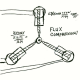Prev: Directional Lights II Next: Point Light Per Pixel

In this section we’ll modify the previous shaders to compute the directional light per pixel. Basically we’re going to split the work between the two shaders, so that some operations are done per pixel.

First lets take a look at the information we receive per vertex:

• normal
• half vector

We have to transform the normal to eye space, and normalize it. This normalized vector is to be interpolated and then sent to the fragment shader so we need to declare varying variables to hold the normals.

We can also perform some computations combining the lights settings with the materials in the vertex shader, hence helping to split the load between the vertex and fragment shader.

```varying vec4 diffuse,ambient;
varying vec3 normal,halfVector;

void main()
{
/* first transform the normal into eye space and
normalize the result */
normal = normalize(gl_NormalMatrix * gl_Normal);

/* pass the halfVector to the fragment shader */
halfVector = gl_LightSource.halfVector.xyz;

/* Compute the diffuse, ambient and globalAmbient terms */
diffuse = gl_FrontMaterial.diffuse * gl_LightSource.diffuse;
ambient = gl_FrontMaterial.ambient * gl_LightSource.ambient;
ambient += gl_LightModel.ambient * gl_FrontMaterial.ambient;
gl_Position = ftransform();

}```

Now for the fragment shader. The same varying variables have to be declared. We have to normalize again the normal. Then we compute the dot product between the interpolated normalized normal and the light direction.

```varying vec4 diffuse,ambient;
varying vec3 normal,halfVector;

void main()
{
vec3 n,halfV,lightDir;
float NdotL,NdotHV;

lightDir = vec3(gl_LightSource.position);

/* The ambient term will always be present */
vec4 color = ambient;
/* a fragment shader can't write a varying variable, hence we need
a new variable to store the normalized interpolated normal */
n = normalize(normal);
/* compute the dot product between normal and ldir */

NdotL = max(dot(n,lightDir),0.0);
....

}```

If the dot product NdotL is greater than zero then we must compute the diffuse component, which is the diffuse setting we received from the vertex shader multiplied by the dot product. We must also compute the specular term. To compute the specular component we must first normalize the halfvector we received from the vertex shader, and also compute the dot product between the normalized halfvector and the normal.

```	...
if (NdotL > 0.0) {
color += diffuse * NdotL;
halfV = normalize(halfVector);
NdotHV = max(dot(n,halfV),0.0);
color += gl_FrontMaterial.specular *
gl_LightSource.specular *
pow(NdotHV, gl_FrontMaterial.shininess);
}

gl_FragColor = color;
}```

The following images show the difference in terms of visual results between computing the lighting per vertex versus per pixel.

 Prev: Directional Lights II Next: Point Light Per Pixel

### 12 Responses to “Directional Light per Pixel”

1.Why does my light angle change when the camera moves?

2.Why n = normalize(normal); if it’s already normalized in the vertex shader with this: normal = normalize(gl_NormalMatrix * gl_Normal); ?

•Hi Ramy,

It is explained in the Normalization Issues page.

3.I’m not sure if you’ll see this, but I was rushing through setting up some per-pixel lighting in my editing tool (lazy bugger I am) I just copy pasted your example code because I couldn’t find my own library of shaders. Anyway I noticed that you declare lightDir in the fragment shader as a float, but try to assign a vec3 to it immediately afterwards.

Thought I’d let you know about that.

•Fixed it. Thanks for the bug report 🙂

•You’re welcome, nothing worse than making a tutorial and making a mistake in it that sends newbies into a frenzy ^^;

4.While this is a great tutorial, I did notice that there are a few syntax errors in your code that prevented compilation.

5.Hello,

Thank you for your execellent tutorial.
I have one question.

In th vertex shader, “nomal” is transformed into eye space and
normalized but that is not necessary. The “normal” is not used in the

Am I missing sometning?

void main()
{
/* first transform the normal into eye space and
normalize the result */
normal = normalize(gl_NormalMatrix * gl_Normal);

varying vec4 diffuse,ambient;
varying vec3 normal,lightDir,halfVector;

void main()
{
… snip
/* a fragment shader can’t write a varying variable, hence we need
a new variable to store the normalized interpolated normal */
n = normalize(normal);

•If the normal is not normalized in the vertex shader then the interpolated normal, in the fragment shader, will not have the right direction.

•I don’t think youre right.
I just checked using my phong shader, and couldn’t notice a single difference when the normal is ALSO normalized in the vertex shader.
••